Paul's Online Notes
Home / Algebra / Graphing and Functions / Lines
Show Mobile Notice Show All Notes Hide All Notes
Mobile Notice
You appear to be on a device with a "narrow" screen width (i.e. you are probably on a mobile phone). Due to the nature of the mathematics on this site it is best views in landscape mode. If your device is not in landscape mode many of the equations will run off the side of your device (should be able to scroll to see them) and some of the menu items will be cut off due to the narrow screen width.

### Section 3.2 : Lines

Let’s start this section off with a quick mathematical definition of a line. Any equation that can be written in the form,

$Ax + By = C$

where we can’t have both $$A$$ and $$B$$ be zero simultaneously is a line. It is okay if one of them is zero, we just can’t have both be zero. Note that this is sometimes called the standard form of the line.

Before we get too far into this section it would probably be helpful to recall that a line is defined by any two points that are on the line. Given two points that are on the line we can graph the line and/or write down the equation of the line. This fact will be used several times throughout this section.

One of the more important ideas that we’ll be discussing in this section is that of slope. The slope of a line is a measure of the steepness of a line and it can also be used to measure whether a line is increasing or decreasing as we move from left to right. Here is the precise definition of the slope of a line.

Given any two points on the line say, $$\left( {{x_1},{y_1}} \right)$$ and $$\left( {{x_2},{y_2}} \right)$$, the slope of the line is given by,

$m = \frac{{{y_2} - {y_1}}}{{{x_2} - {x_1}}}$

In other words, the slope is the difference in the $$y$$ values divided by the difference in the $$x$$ values. Also, do not get worried about the subscripts on the variables. These are used fairly regularly from this point on and are simply used to denote the fact that the variables are both $$x$$ or $$y$$ values but are, in all likelihood, different.

When using this definition do not worry about which point should be the first point and which point should be the second point. You can choose either to be the first and/or second and we’ll get exactly the same value for the slope.

There is also a geometric “definition” of the slope of the line as well. You will often hear the slope as being defined as follows,

$m = \frac{{{\mbox{rise}}}}{{{\mbox{run}}}}$

The two definitions are identical as the following diagram illustrates. The numerators and denominators of both definitions are the same.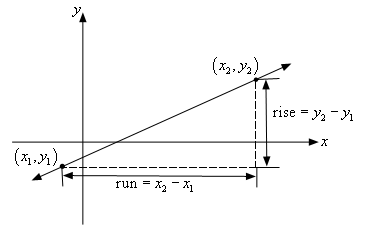Note as well that if we have the slope (written as a fraction) and a point on the line, say $$\left( {{x_1},{y_1}} \right)$$, then we can easily find a second point that is also on the line. Before seeing how this can be done let’s take the convention that if the slope is negative we will put the minus sign on the numerator of the slope. In other words, we will assume that the rise is negative if the slope is negative. Note as well that a negative rise is really a fall.

So, we have the slope, written as a fraction, and a point on the line, $$\left( {{x_1},{y_1}} \right)$$. To get the coordinates of the second point, $$\left( {{x_2},{y_2}} \right)$$ all that we need to do is start at $$\left( {{x_1},{y_1}} \right)$$ then move to the right by the run (or denominator of the slope) and then up/down by rise (or the numerator of the slope) depending on the sign of the rise. We can also write down some equations for the coordinates of the second point as follows,

\begin{align*}{x_2} & = {x_1} + \,\,{\mbox{run}}\\ {y_2} & = {y_1} + \,\,{\mbox{rise}}\end{align*}

Note that if the slope is negative then the rise will be a negative number.

Let’s compute a couple of slopes.

Example 1 Determine the slope of each of the following lines. Sketch the graph of each line.
1. The line that contains the two points $$\left( { - 2, - 3} \right)$$ and $$\left( {3,1} \right)$$.
2. The line that contains the two points $$\left( { - 1,5} \right)$$ and $$\left( {0, - 2} \right)$$.
3. The line that contains the two points $$\left( { - 3,2} \right)$$ and $$\left( {5,2} \right)$$.
4. The line that contains the two points $$\left( {4,3} \right)$$ and $$\left( {4, - 2} \right)$$.
Show All Solutions Hide All Solutions
Show Discussion

Okay, for each of these all that we’ll need to do is use the slope formula to find the slope and then plot the two points and connect them with a line to get the graph.

a The line that contains the two points $$\left( { - 2, - 3} \right)$$ and $$\left( {3,1} \right)$$. Show Solution

Do not worry which point gets the subscript of 1 and which gets the subscript of 2. Either way will get the same answer. Typically, we’ll just take them in the order listed. So, here is the slope for this part.

$m = \frac{{1 - \left( { - 3} \right)}}{{3 - \left( { - 2} \right)}} = \frac{{1 + 3}}{{3 + 2}} = \frac{4}{5}$

Be careful with minus signs in these computations. It is easy to lose track of them. Also, when the slope is a fraction, as it is here, leave it as a fraction. Do not convert to a decimal unless you absolutely have to.

Here is a sketch of the line.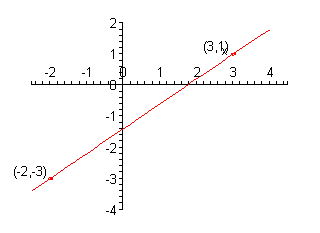Notice that this line increases as we move from left to right.

b The line that contains the two points $$\left( { - 1,5} \right)$$ and $$\left( {0, - 2} \right)$$. Show Solution

Here is the slope for this part.

$m = \frac{{ - 2 - 5}}{{0 - \left( { - 1} \right)}} = \frac{{ - 7}}{1} = - 7$

Again, watch out for minus signs. Here is a sketch of the graph.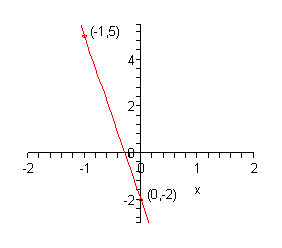This line decreases as we move from left to right.

c The line that contains the two points $$\left( { - 3,2} \right)$$ and $$\left( {5,2} \right)$$. Show Solution

Here is the slope for this line.

$m = \frac{{2 - 2}}{{5 - \left( { - 3} \right)}} = \frac{0}{8} = 0$

We got a slope of zero here. That is okay, it will happen sometimes. Here is the sketch of the line.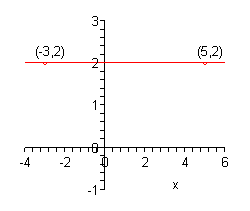In this case we’ve got a horizontal line.

d The line that contains the two points $$\left( {4,3} \right)$$ and $$\left( {4, - 2} \right)$$. Show Solution

The final part. Here is the slope computation.

$m = \frac{{ - 2 - 3}}{{4 - 4}} = \frac{{ - 5}}{0} = {\mbox{undefined}}$

In this case we get division by zero which is undefined. Again, don’t worry too much about this it will happen on occasion. Here is a sketch of this line.This final line is a vertical line.

We can use this set of examples to see some general facts about lines.

First, we can see from the first two parts that the larger the number (ignoring any minus signs) the steeper the line. So, we can use the slope to tell us something about just how steep a line is.

Next, we can see that if the slope is a positive number then the line will be increasing as we move from left to right. Likewise, if the slope is a negative number then the line will decrease as we move from left to right.

We can use the final two parts to see what the slopes of horizontal and vertical lines will be. A horizontal line will always have a slope of zero and a vertical line will always have an undefined slope.

We now need to take a look at some special forms of the equation of the line.

We will start off with horizontal and vertical lines. A horizontal line with a $$y$$-intercept of $$b$$ will have the equation,

$\require{bbox} \bbox[2pt,border:1px solid black]{{y = b}}$

Likewise, a vertical line with an $$x$$-intercept of $$a$$ will have the equation,

$\require{bbox} \bbox[2pt,border:1px solid black]{{x = a}}$

So, if we go back and look that the last two parts of the previous example we can see that the equation of the line for the horizontal line in the third part is

$y = 2$

while the equation for the vertical line in the fourth part is

$x = 4$

The next special form of the line that we need to look at is the point-slope form of the line. This form is very useful for writing down the equation of a line. If we know that a line passes through the point $$\left( {{x_1},{y_1}} \right)$$ and has a slope of $$m$$ then the point-slope form of the equation of the line is,

$\require{bbox} \bbox[2pt,border:1px solid black]{{y - {y_1} = m\left( {x - {x_1}} \right)}}$

Sometimes this is written as,

$\require{bbox} \bbox[2pt,border:1px solid black]{{y = {y_1} + m\left( {x - {x_1}} \right)}}$

The form it’s written in usually depends on the instructor that is teaching the class.

As stated earlier this form is particularly useful for writing down the equation of a line so let’s take a look at an example of this.

Example 2 Write down the equation of the line that passes through the two points $$\left( { - 2,4} \right)$$ and $$\left( {3, - 5} \right)$$.
Show Solution

At first glance it may not appear that we’ll be able to use the point-slope form of the line since this requires a single point (we’ve got two) and the slope (which we don’t have). However, that fact that we’ve got two points isn’t really a problem; in fact, we can use these two points to determine the missing slope of the line since we do know that we can always find that from any two points on the line.

So, let’s start off my finding the slope of the line.

$m = \frac{{ - 5 - 4}}{{3 - \left( { - 2} \right)}} = - \frac{9}{5}$

Now, which point should we use to write down the equation of the line? We can actually use either point. To show this we will use both.

First, we’ll use$$\left( { - 2,4} \right)$$. Now that we’ve gotten the point all that we need to do is plug into the formula. We will use the second form.

$y = 4 - \frac{9}{5}\left( {x - \left( { - 2} \right)} \right) = 4 - \frac{9}{5}\left( {x + 2} \right)$

Now, let’s use $$\left( {3, - 5} \right)$$.

$y = - 5 - \frac{9}{5}\left( {x - 3} \right)$

Okay, we claimed that it wouldn’t matter which point we used in the formula, but these sure look like different equations. It turns out however, that these really are the same equation. To see this all that we need to do is distribute the slope through the parenthesis and then simplify.

Here is the first equation.

\begin{align*}y & = 4 - \frac{9}{5}\left( {x + 2} \right)\\ & = 4 - \frac{9}{5}x - \frac{{18}}{5}\\ & = - \frac{9}{5}x + \frac{2}{5}\end{align*}

Here is the second equation.

\begin{align*}y & = - 5 - \frac{9}{5}\left( {x - 3} \right)\\ & = - 5 - \frac{9}{5}x + \frac{{27}}{5}\\ & = - \frac{9}{5}x + \frac{2}{5}\end{align*}

So, sure enough they are the same equation.

The final special form of the equation of the line is probably the one that most people are familiar with. It is the slope-intercept form. In this case if we know that a line has slope $$m$$ and has a $$y$$-intercept of $$\left( {0,b} \right)$$ then the slope-intercept form of the equation of the line is,

$y = mx + b$

This form is particularly useful for graphing lines. Let’s take a look at a couple of examples.

Example 3 Determine the slope of each of the following equations and sketch the graph of the line.
1. $$2y - 6x = - 2$$
2. $$3y + 4x = 6$$
Show All Solutions Hide All Solutions
Show Discussion

Okay, to get the slope we’ll first put each of these in slope-intercept form and then the slope will simply be the coefficient of the $$x$$ (including sign). To graph the line we know the $$y$$-intercept of the line, that’s the number without an $$x$$ (including sign) and as discussed above we can use the slope to find a second point on the line. At that point there isn’t anything to do other than sketch the line.

a $$2y - 6x = - 2$$ Show Solution

First solve the equation for $$y$$. Remember that we solved equations like this back in the previous chapter.

\begin{align*}2y = 6x - 2\\ & y = 3x - 1\end{align*}

So, the slope for this line is 3 and the $$y$$-intercept is the point $$\left( {0, - 1} \right)$$. Don’t forget to take the sign when determining the $$y$$-intercept. Now, to find the second point we usually like the slope written as a fraction to make it clear what the rise and run are. So,

$m = 3 = \frac{3}{1} = \frac{{{\mbox{rise}}}}{{{\mbox{run}}}}\hspace{0.25in} \Rightarrow \hspace{0.25in}{\mbox{rise}} = 3,\,\,\,\,{\mbox{run}} = 1$

The second point is then,

${x_2} = 0 + 1 = 1\hspace{0.25in}\hspace{0.25in}{y_2} = - 1 + 3 = 2\hspace{0.25in}\hspace{0.25in} \Rightarrow \hspace{0.25in}\left( {1,2} \right)$

Here is a sketch of the graph of the line.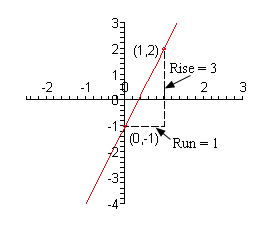b $$3y + 4x = 6$$ Show Solution

Again, solve for $$y$$.

\begin{align*}3y = - 4x + 6\\ & y = - \frac{4}{3}x + 2\end{align*}

In this case the slope is $$- \frac{4}{3}$$ and the $$y$$-intercept is $$\left( {0,2} \right)$$. As with the previous part let’s first determine the rise and the run.

$m = - \frac{4}{3} = \frac{{ - 4}}{3} = \frac{{{\mbox{rise}}}}{{{\mbox{run}}}}\hspace{0.25in}\hspace{0.25in} \Rightarrow \hspace{0.25in}{\mbox{rise}} = - 4,\,\,\,{\mbox{run}} = 3$

Again, remember that if the slope is negative make sure that the minus sign goes with the numerator. The second point is then,

${x_2} = 0 + 3 = 3\hspace{0.25in}\hspace{0.25in}{y_2} = 2 + \left( { - 4} \right) = - 2\hspace{0.25in}\hspace{0.25in} \Rightarrow \hspace{0.25in}\left( {3, - 2} \right)$

Here is the sketch of the graph for this line.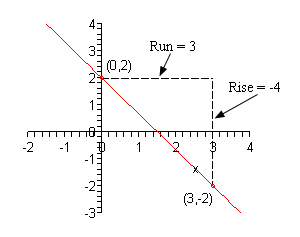The final topic that we need to discuss in this section is that of parallel and perpendicular lines. Here is a sketch of parallel and perpendicular lines.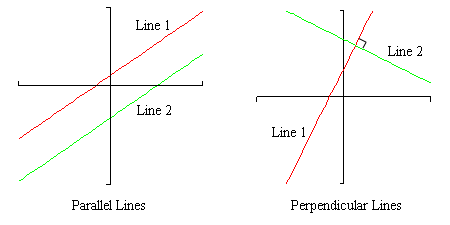Suppose that the slope of Line 1 is $${m_1}$$ and the slope of Line 2 is $${m_2}$$. We can relate the slopes of parallel lines and we can relate slopes of perpendicular lines as follows.

\begin{align*} & {\mbox{parallel : }} {m_1} = {m_2}\\ & {\mbox{perpendicular : }}{m_1}{m_2} = - 1\,\,\,{\mbox{or }}{m_2} = - \frac{1}{{{m_1}}}\end{align*}

Note that there are two forms of the equation for perpendicular lines. The second is the more common and in this case we usually say that $${m_2}$$ is the negative reciprocal of $${m_1}$$.

Example 4 Determine if the line that passes through the points $$\left( { - 2, - 10} \right)$$ and $$\left( {6, - 1} \right)$$ is parallel, perpendicular or neither to the line given by $$7y - 9x = 15$$.
Show Solution

Okay, in order to do answer this we’ll need the slopes of the two lines. Since we have two points for the first line we can use the formula for the slope,

${m_1} = \frac{{ - 1 - \left( { - 10} \right)}}{{6 - \left( { - 2} \right)}} = \frac{9}{8}$

We don’t actually need the equation of this line and so we won’t bother with it.

Now, to get the slope of the second line all we need to do is put it into slope-intercept form.

\begin{align*}7y & = 9x + 15\\ y & = \frac{9}{7}x + \frac{{15}}{7}\hspace{0.25in}\hspace{0.25in} \Rightarrow \hspace{0.25in}\hspace{0.25in}{m_2} = \frac{9}{7}\end{align*}

Okay, since the two slopes aren’t the same (they’re close, but still not the same) the two lines are not parallel. Also,

$\left( {\frac{9}{8}} \right)\left( {\frac{9}{7}} \right) = \frac{{81}}{{56}} \ne - 1$

so the two lines aren’t perpendicular either.

Therefore, the two lines are neither parallel nor perpendicular.

Example 5 Determine the equation of the line that passes through the point $$\left( {8,2} \right)$$ and is,
1. parallel to the line given by $$10y + 3x = - 2$$
2. perpendicular to the line given by $$10y + 3x = - 2$$.
Show All Solutions Hide All Solutions
Show Discussion

In both parts we are going to need the slope of the line given by $$10y + 3x = - 2$$ so let’s actually find that before we get into the individual parts.

\begin{align*}10y & = - 3x - 2\\ y & = - \frac{3}{{10}}x - \frac{1}{5}\hspace{0.25in} \Rightarrow \hspace{0.25in}{m_1} = - \frac{3}{{10}}\end{align*}

Now, let’s work the example.

a parallel to the line given by $$10y + 3x = - 2$$ Show Solution

In this case the new line is to be parallel to the line given by $$10y + 3x = - 2$$ and so it must have the same slope as this line. Therefore, we know that,

${m_2} = - \frac{3}{{10}}$

Now, we’ve got a point on the new line, $$\left( {8,2} \right)$$, and we know the slope of the new line, $$- \frac{3}{{10}}$$, so we can use the point-slope form of the line to write down the equation of the new line. Here is the equation,

\begin{align*}y & = 2 - \frac{3}{{10}}\left( {x - 8} \right)\\ & = 2 - \frac{3}{{10}}x + \frac{{24}}{{10}}\\ & = - \frac{3}{{10}}x + \frac{{44}}{{10}}\\ y & = - \frac{3}{{10}}x + \frac{{22}}{5}\end{align*}

b perpendicular to the line given by $$10y + 3x = - 2$$. Show Solution

For this part we want the line to be perpendicular to $$10y + 3x = - 2$$ and so we know we can find the new slope as follows,

${m_2} = - \frac{1}{{ - \frac{3}{{10}}}} = \frac{{10}}{3}$

Then, just as we did in the previous part we can use the point-slope form of the line to get the equation of the new line. Here it is,

\begin{align*}y & = 2 + \frac{{10}}{3}\left( {x - 8} \right)\\ & = 2 + \frac{{10}}{3}x - \frac{{80}}{3}\\ y & = \frac{{10}}{3}x - \frac{{74}}{3}\end{align*}